﻿ Use of the Triangular Fuzzy Numbers for Student Assessment

### Use of the Triangular Fuzzy Numbers for Student Assessment

Michael Gr. Voskoglou

## Use of the Triangular Fuzzy Numbers for Student Assessment

Michael Gr. VoskoglouDepartment of Mathematical Sciences, School of Technological Applications, Graduate Technological Educational Institute (T. E. I.) of Western Greece, Patras, Greece

### Abstract

The social demand not only to educate, but also to classify students according to their qualifications, makes the student assessment one of the most important components of the educational process. Fuzzy logic, due to its nature of characterizing a case with multiple values, offers rich resources for the ssessment purposes. This gave us several times in past the impulse to apply principles of fuzzy logic for assessing human skills using as tools the corresponding system’s total uncertainty, the COG defuzzification technique and recently developed variations of it. In the present paper we use the Triangular Fuzzy Numbers (TFNs) as an alternative tool for the same purpose and we compare this approach with the assessment methods of the bivalent and fuzzy logic that we have already used in earlier works. Our ambition for this paper is to be easily understood by the non expert on fuzzy logic reader and therefore the TFNs and the arithmetic operations defined on them are presented in a simple way, by giving examples and by avoiding, as much as we can, the excessive mathematical severity.

• Michael Gr. Voskoglou. Use of the Triangular Fuzzy Numbers for Student Assessment. American Journal of Applied Mathematics and Statistics. Vol. 3, No. 4, 2015, pp 146-150. http://pubs.sciepub.com/ajams/3/4/2
• Voskoglou, Michael Gr.. "Use of the Triangular Fuzzy Numbers for Student Assessment." American Journal of Applied Mathematics and Statistics 3.4 (2015): 146-150.
• Voskoglou, M. G. (2015). Use of the Triangular Fuzzy Numbers for Student Assessment. American Journal of Applied Mathematics and Statistics, 3(4), 146-150.
• Voskoglou, Michael Gr.. "Use of the Triangular Fuzzy Numbers for Student Assessment." American Journal of Applied Mathematics and Statistics 3, no. 4 (2015): 146-150.

 Import into BibTeX Import into EndNote Import into RefMan Import into RefWorks

1
Prev Next

### 1. Introduction

They are often situations in everyday life in which definitions have not clear boundaries; e.g. this happens when we speak about the “high mountains” of a country, the “good players” of a football team, etc. The fuzzy sets theory was created in response to have a mathematical representation of such kind of situations. The notion of a fuzzy set initiated by Zadeh in 1965  is defined as follows:

Definition 1: Let U denote the universal set of the discourse. Then a fuzzy set A on U (or otherwise a fuzzy subset of U), , is defined in terms of the membership function mΑ that assigns to each element of U a real value from the interval [0,1]. In more specific terms a fuzzy set A in U can be written as a set of ordered pairs in the form Α = {(x, mΑ(x)): x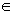U}{1}, where mΑ : U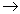[0,1].

The value mΑ(x), called the membership degree (or grade) of x in A, expresses the degree to which x verifies the characteristic property of A. Thus, the nearer is the value mΑ(x) to 1, the higher is the membership degree of x in A. The methods of choosing the proper membership function are empiric, based usually on statistical data of experiments performed with samples of the population under study. However, a necessary condition for the creditability of a fuzzy set in representing a real situation is that the criteria of the choice of the corresponding membership function are compatible to the common logic.

Obviously each classical (crisp) subset A of U can be considered as a fuzzy subset of U, with mΑ(x)=1 if xU and mΑ(x)=0 if x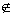U. Most of the concepts of classical (crisp) sets can be extended in terms of the above definition to fuzzy sets.

Despite the fact that both operate over the same numeric range [0, 1], fuzzy set theory is distinct from probability theory. For example, the probabilistic approach yields the natural language statement “there is an 85% chance that Mary is tall”, while the fuzzy terminology corresponds to the expression “Mary’s degree of membership within the set of tall people is 0.85”. The semantic difference is significant: The first view supposes that Mary is or is not tall (still caught in the law of the Excluded Middle); it is just that we only have a 85% chance of knowing in which set she is in. In contrast, fuzzy terminology supposes that Mary is “more or less” tall, or some other term corresponding to the value of 0.85. For general facts on fuzzy sets we refer to the book .

Fuzzy logic, the development of which is based on fuzzy sets theory , provides a rich and meaningful addition to standard Boolean logic. Unlike Boolean logic, which has only two states, true or false, fuzzy logic deals with truth values which range continuously from 0 to 1. Thus something could be half true 0.5 or very likely true 0.9 or probably not true 0.1, etc. In this way fuzzy logic allows one to express knowledge in a rule format that is close to a natural language expression and therefore it opens the door to construction of mathematical solutions of computational problems which are inherently imprecisely defined.

The assessment of a system’s effectiveness (i.e. of the degree of attainment of its targets) with respect to an action performed within the system (e.g. problem-solving, decision making, learning performance, etc) is a very important task that enables the correction of the system’s weaknesses resulting to the improvement of its general performance. The assessment methods that are commonly used in practice are based on the principles of the classical, bivalent logic (yes-no). However, there are cases where a crisp characterization is not probably the proper one for an assessment. For example, a teacher is frequently not sure about a particular numerical grade characterizing a student’s performance.

Fuzzy logic, due to its nature of characterizing a case with multiple values, offers wider and richer resources covering such kind of cases. This gave as several times in the past the impulse to apply principles of fuzzy logic for the assessment of human or machine (in case of CBR systems) skills using as tools the corresponding system’s total uncertainty (e.g. see  and its relevant references, , etc), the Center of Gravity (COG) defuzzification technique (e.g. see [6, 14], etc) as well as the recently developed variations of this technique of the Triangular (e.g. see [7, 8, 13], etc) and of the Trapezoidal (e.g. see [9, 14], etc) fuzzy assessment models. The above fuzzy methods, although they can be used for individual assessment as well , they are more appropriate for accessing the overall performance of a group of individuals (or objects) sharing common characteristics (e.g. students, players, CBR systems, etc). For some more details about these methods see also Section 4.

In the present paper we shall use the Triangular Fuzzy Numbers (TFNs) for assessing student skills. In contrast to the above mentioned fuzzy assessment methods, this approach is more appropriate for individual assessment. However, we shall adapt it to use it as a tool for group assessment too.

The rest of the paper is organized as follows: In Section 2 we present the notion of Fuzzy Numbers (FN), while in Section 3 we present the TFNs and the arithmetic operations defined among them. In Section 4 we describe how one can use the TFNs for assessing student skills and we discuss the advantages and disadvantages of this method with respect to the alternative fuzzy assessment methods applied in earlier papers (see above) Finally, Section 5 is devoted to our conclusion and a brief discussion on the perspectives of future research on the subject.

### 2. Fuzzy Numbers

A Fuzzy Number (FN) is a special form of fuzzy sets on the set R of real numbers. FNs play a fundamental role in fuzzy mathematics, analogous to the role played by the ordinary numbers in classical mathematics. For general facts on FNs we refer to Chapter 3 of the book , which is written in Greek language, and also to the books [3, 4].

For the better understanding of the notion of a FN (by those not familiar to it) we shall start with the following three introductory definitions:

Definition 2: A fuzzy set A on U with membership function y=m(x) is said to be normal, if there exists x in U, such that m(x) = 1.

Definition 3: Let A be as in definition 2, and let x be a real number of the interval [0, 1]. Then the x-cut of A, denoted by Ax, is defined to be the set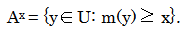Definition 4: A fuzzy set A on R is said to be convex, if its x-cuts Ax are ordinary closed real intervals, for all x in [0, 1].

For example, for the fuzzy set A whose membership function’s graph is represented in Figure 1, we observe that A0.4 = [5, 8.5]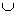[11, 13] and therefore A is not a convex fuzzy set.Download asVeiw figureFigures index
Figure 1. Graph of a non convex fuzzy set

We are ready now to give the definition of a FN:

Definition 5: A fuzzy number is a normal and convex fuzzy set A on R with a piecewise continuous membership function.

Figure 2 represents the graph of a FN expressing the fuzzy concept: The real number x is approximately equal to 5”. We observe that the membership function of this FN takes constantly the value 0 outside the interval [0, 10].Download asVeiw figureFigures index
Figure 2. Graph of a fuzzy number

Since the x-cuts Ax of a FN A are closed real intervals, we can write Ax = [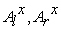] for each x in [0,1], whereare real numbers depending on x.

The following statement defines a partial order in the set of all FNs:

Definition 6: Given the FNs A and B we write A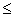B (or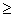) if, and only if,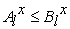and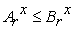(or) for all x in [0, 1]. Two FNs for which the above relation holds are called comparable, otherwise they are called non comparable.

### 3. Triangular Fuzzy Numbers (TFNs)

3.1. Definition of TFNs

Let us consider the FN, say A, of Figure 3 representing the same fuzzy concept with the FN of Figure 2. We observe that the membership function y=m(x) of A takes constantly the value 0, if x is outside the interval [0, 10], while its graph in the interval [0, 10] is the union of two straight line segments forming a triangle with the OX axis. More explicitly, we have y = m(x) =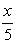, if x is in [0, 5], and y = m(x) =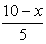, if x is in [5, 10]. This is a typical example of a TFN, symbolized by A= (0, 5, 10).Download asVeiw figureFigures index
Figure 3. Graph of the TFN (0, 5, 10)

In general, the definition of a TFN is given as follows:

Definition 7: Let a, b and c be real numbers with a < b < c. Then the Triangular Fuzzy Number (TFN) A = (a, b, c) is the FN with membership function: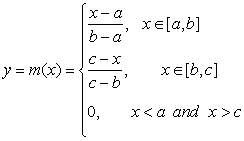Obviously we have that m(b)=1, while b need not be in the “middle” of a and c.

It is well known that for a TFN A = (a, b, c), the x-cut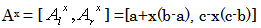(1)
3.2. Arithmetic Operations between TFNs

The basic arithmetic operations between FNs are performed in general in two alternative ways:

i) With the help of the x-cuts of the corresponding FNs, which, as we have already seen, are ordinary closed intervals of R.

Therefore, according to this approach, the Fuzzy Arithmetic is actually based on the arithmetic of the real intervals.

ii) By applying the Zadeh’s extension principle (see Section 1.4, p.20 of ), which provides the means for any function f mapping the crisp set X to the crisp set Y to be generalized so that to map fuzzy subsets of X to fuzzy subsets of Y.

In practice the above two general methods of the fuzzy arithmetic, requiring laborious calculations, are rarely used in applications, where the utilization of simpler forms of FNs is preferred, including the TFNs.

It can be shown that the above two general methods lead to the following simple rules for the addition and subtraction of TFNs:

Let A = (a, b, c) and B = (a1, b1, c1) be two TFNs. Then

•  The sum A + B = (a+a1, b+b1, c+c1).

•  The difference A - B = A + (-B) = (a-c1, b-b1, c-a1), where –B = (-c1, -b1, -a1) is defined to be the opposite of B.

In other words, the opposite of a TFN, as well as the sum and the difference of two TFNs are also TFNs.

On the contrary, the product and the quotient of two TFNs, although they are FNs, they are not always TFNs. However, in the special case where a, b, c, a1, b1, c1 are in R+, it can be shown that the fuzzy operations of multiplication and division of TFNs can be approximately performed by the rules:

•  The product A . B = (aa1, bb1, cc1).

•  The quotient A : B = A . B-1 = (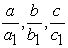), where B-1 = (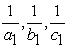) is defined to be the inverse of B.

In other words, in R+ the inverse of a TFN, as well as the product and the division of two TFNs can be approximately considered to be TFNs too.

Further, one can define the following two scalar operations:

•  k + A= (k+a, k+b, k+c), kR

•  kA = (ka, kb, kc), if k>0 and kA = (kc, kb, ka), if k<0.

### 4. Use of the TFNs for Student Assessment

The social demand not only to educate, but also to classify students according to their qualifications, makes the student assessment one of the most important components of the educational process. On the other hand, the teacher, obtaining through the student assessment an overall view of his (her) students’ progress, is helped to suitably adapt his (her) teaching methods and plans aiming to the best possible result.

In this section we shall use the TFNs as an alternative tool for the student assessment. This approach enables the teacher to deal better with the ambiguous cases of students, for which he (she) is not absolutely sure (as it frequently happens) for a numerical grade representing exactly their progress. For this, we reconsider the following example, firstly presented in :

EXAMPLE: The students of two different Departments of the School of Management and Economics of the Graduate Technological Educational Institute (T. E. I.) of Western Greece achieved the following scores (in a climax from 0 to 100) at their common progress exam in the course “Mathematics for Economists I”:

Department 1 (D1): 100(5 times), 99(3), 98(10), 95(15), 94(12), 93(1), 92 (8), 90(6), 89(3), 88(7), 85(13), 82(4), 80(6), 79(1), 78(1), 76(2), 75(3), 74(3), 73(1), 72(5), 70(4), 68(2), 63(2), 60(3), 59(5), 58(1), 57(2), 56(3), 55(4), 54(2), 53(1), 52(2), 51(2), 50(8), 48(7), 45(8), 42(1), 40(3), 35(1).

Department 2 (D2 : 100(7), 99(2), 98(3), 97(9), 95(18), 92(11), 91(4), 90(6), 88(12), 85(36), 82(8), 80(19), 78(9), 75(6), 70(17), 64(12), 60(16), 58(19), 56(3), 55(6), 50(17), 45(9), 40(6).

4.1. Summary of Our Previous Research (for Details See )

Calculating the means of the above scores, one approximately finds the value 76.006 for D1 and the value 75.09 for D2 respectively, showing that D1 demonstrated a slightly better mean performance than D2.

Further, in  we introduced the set U = {A, B, C, D, F} of linguistic labels corresponding to the above scores as follows: A (85-100) = excellent, B (84-75) = very good, C (74-60) = good, D (59-50) = fair and F (<50) = unsatisfactory.

Then, we calculated in  the GPA index for each department by the formula GPA=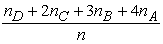(e.g. cf. ), where nx denotes the number of students of the department whose scores correspond to the linguistic label (grade) x in U and we found the value GPA = 2,529 for both departments. The value of GPA, always in the frames of the bivalent logic, characterizes the quality performance of each department, because in the above formula higher coefficients are attached to the higher scores.

Next, in  we applied three different fuzzy methods for the student assessment: First, expressing the two departments as fuzzy sets in U (the membership function was defined by m(x) =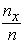for each department), we calculated the total (possibilistic) uncertainty in each case and we found the values 0.259 for D1 and 0.934 for D2 respectively. This shows that D1 demonstrated a considerably better (mean) performance than D2. Further, the application of the COG defuzzification technique, as well as of the TRFAM showed that (in contrast to the mean performance) D2 demonstrated a slightly better quality performance than D1.

Finally in , the differences appeared in the results obtained by applying the above (five in total) traditional and fuzzy assessment methods, were adequately explained and justified through the nature of each method. This provided a very strong indication for the creditability of the above three innovative fuzzy assessment methods.

4.2. Use of the TFNs for Individual Student Assessment

Let us now come to the main purpose of this section, which is the use of TFNs as an. alternative tool for student assessment, For this, we assign to each linguistic label (grade) of the set U considered in Section TFN (denoted by the same letter) as follows: A= (85, 92.5, 100), B = (75, 79.5, 84), C = (60, 67, 74), D= (50, 54.5, 59) and F = (0, 24.5, 49).

The process followed for the above assignment is quite obvious. Namely, the middle entry of each TFN is equal to the mean value of the student scores that we have previously attached to the corresponding linguist label (grade). In this way a TFN corresponds to each student assessing his (her) individual performance.

Notice that in , as an alternative individual student assessment method, an ordered triple of fuzzy linguistic labels was assigned to each student. It was shown also in  that this approach is equivalent with the A. Jones method  of assessing a student’s knowledge in terms of his (her) fuzzy deviation with respect to the teacher. However, the method with the TFNs presented here is more comprehensive, since it treats the (fuzzy) individual student assessment numerically.

4.3. Use of the TFNs for Assessing the Overall Performance of the Two Departments

Next, we are going to adapt the individual student assessment presented in Section 4.2 in order to be used for assessing in our Example the overall performance of each department in terms of the TFNs, For this, we give the following definition:

Definition 8: Let Ai (ai, bi, ci) be n TFNs, where n is a non negative integer, n2. Then we define the mean value of the above TFNs to be the TFN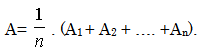We now form Table 1 summarizing the students’ performance in terms of the TFNs defined in Section 4.2 as follows:

#### Table 1. Students’ performance in terms of the TFNsDownload asVeiw tableTables index

In Table 1 we actually have 170 TFNs representing the progress of the students of D1 and 255 TFNs representing the progress of the students of D2. Therefore, it is logical to accept that the overall performance of each department is given by the corresponding mean value of the above TFNs.

For simplifying our symbols, let us denote the above means by the letter of the corresponding department. Then, making straightforward calculations, we find that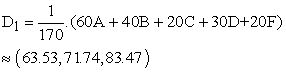and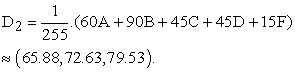On comparing the above two TFNs with the linguistic labels (grades), defined in Section 4.1 with respect to the students’ scores, we observe that the overall performance of the two departments can be characterized from good (C) to very good (B).

Further, applying formula (1) of Section 3.1 one finds that the x-cuts of the two TFNs are D1x = [63.53+8.21x, 83.47-11.73x] and D2x = [65.88+6.75x, 79.53-6.9x] respectively. But 63.53+8.21x65.88+6.75x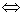1.46x2.35x1.61, which is true, since x is in [0, 1]. On the contrary, 83.47-11.73x79.53-6.9x3.944.83x0.82x, which is not true for all the values of x. Therefore, according to the Definition 6 of Section 2 the TFNs D1 and D2 are not comparable, which means that one can not decide which of the two departments demonstrates the better performance. This is the main disadvantage of the student assessment with the use of TFNs with respect to the other assessment methods reported in Section 4.1.

### 5. Conclusion

In the present paper we used the TFNs as a tool for student assessment, The main advantage of this approach is that in case of individual assessment leads to a numerical result, which is more comprehensive than the qualitative results obtained in earlier works by applying alternative fuzzy assessment methods. On the contrary, in case of group assessment this method leads only to a linguistic characterization of the corresponding group’s overall performance and it is not always sufficient to compare the performances of two different groups, as our fuzzy assessment methods applied in earlier works do. This is due to the fact that the inequality between FNs defines on them a relation of partial order only.

Our method of using the TFNs for student assessment has a general character and therefore it could be utilized in future for assessing other human (or machine) activities too. Further, the utilization of other types of FNs as assessment tools could be of particular interest. For example, the trapezoidal FNs could be used in case where our assessment objects are characterized (in terms of the bivalent logic) by a numerical value lying in a real interval. All the above will be targets of our future research on the subject.

### Note

1. Notice that there are also alternative methods in use for the symbolic representation of a fuzzy set. In fact, if U is a finite set then A can be written as a symbolic sum of the form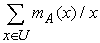, if U is a denumerable set then A can be written as a symbolic infinite sum of the form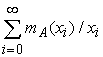, while if U has the power of the continuous then A is usually written as a symbolic integral of the form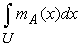. Notice also that, for reasons of simplicity, many authors identify the notion of a fuzzy set with its membership function.

### References

  Grade Point Average Assessment, available in the Web at: http://www.swinburne.edu.au/student-administration/assessment/gpa.html , visited October 15, 2014.In article  Jones, A., Kaufmman, A. & Zimmerman, H. J. (Eds.), Fuzzy Sets: Theory and Applications, NATO ASI Series, Series C: Mathematical and Physical Sciences, Vol. 177, Reidel Publishing Company, Dordrecht, Holland.In article  Hanss, M., Applied Fuzzy Arithmetic, An Introduction with Engineering Applications, Springer-Verlag, Berlin-Heidelberg, 2005.In article  Kaufmann, A. & Gupta, M., Introduction to Fuzzy Arithmetic, Van Nostrand Reinhold Company, , New York, 1991.In article  Klir, G. J., Folger, T. A., Fuzzy Sets, Uncertainty and Information, London, Prentice-Hall, London, 1988.In article  Subbotin, I. Ya., Badkoobehi, H. , Bilotskii, N. N., Application of fuzzy logic to learning assessment. Didactics of Mathematics: Problems and Investigations, 22, 38-41, 2004.In article  Subbotin, I. Ya., Bilotskii, N. N., Triangular fuzzy logic model for learning assessment, Didactics of Mathematics: Problems and Investigations, 41, 84-88, 2014.In article  Subbotin, I. Ya, & Voskoglou, M. Gr., A Triangular Fuzzy Model for Assessing Critical Thinking Skills, International Journal of Applications of Fuzzy Sets and Artificial intelligence, 4, 173 -186, 2014.In article  Subbotin, I. Ya. , Trapezoidal Fuzzy Logic Model for Learning Assessment, arXiv: 1407.0823 [math.GM], submitted on July 1, 2014.In article  Theodorou, J., Introduction to Fuzzy Logic, Tzolas Publications, Thessaloniki, Greece, 2010 (in Greek language).In article  Voskoglou, M. Gr., Stochastic and fuzzy models in Mathematics Education, Artificial Intelligence and Management, Saarbrucken - Germany, Lambert Academic Publishing, 2011.In article  Voskoglou, M. Gr., Assessing Students Individual Problem Solving Skills, International Journal of Applications of Fuzzy Sets and Artificial Intelligence, 3, 39-49, 2013.In article  Voskoglou, M. Gr. & Subbotin, I. Ya., An Application of the Triangular Fuzzy Model to Assessment of Analogical Reasoning Skills, American Journal of Applied Mathematics and Statistics, 3-1, 1-6, 2015.In article  Voskoglou, M. Gr., Fuzzy methods for student assessment, International Journal of Education and Information Technology, 1(1), 20-28, 2015In article  Zadeh, L. A., Fuzzy Sets, Information and Control, 8, 338-353, 1965.In article View Article  Zadeh, L. A., The concept of a linguistic variable and its application to approximate reasoning II, Information Sciences, 8(4), 301-357, 1975.In article View Article